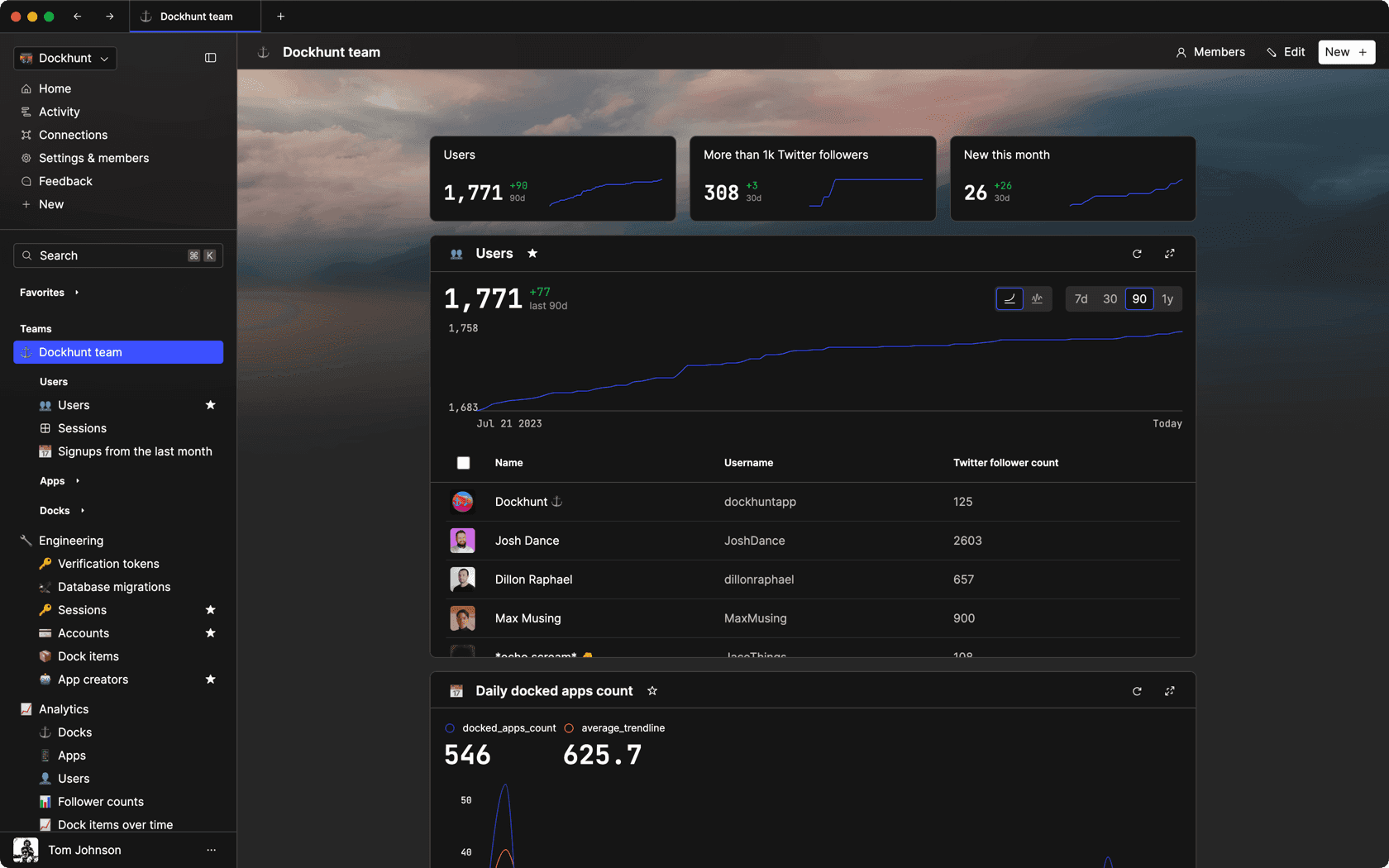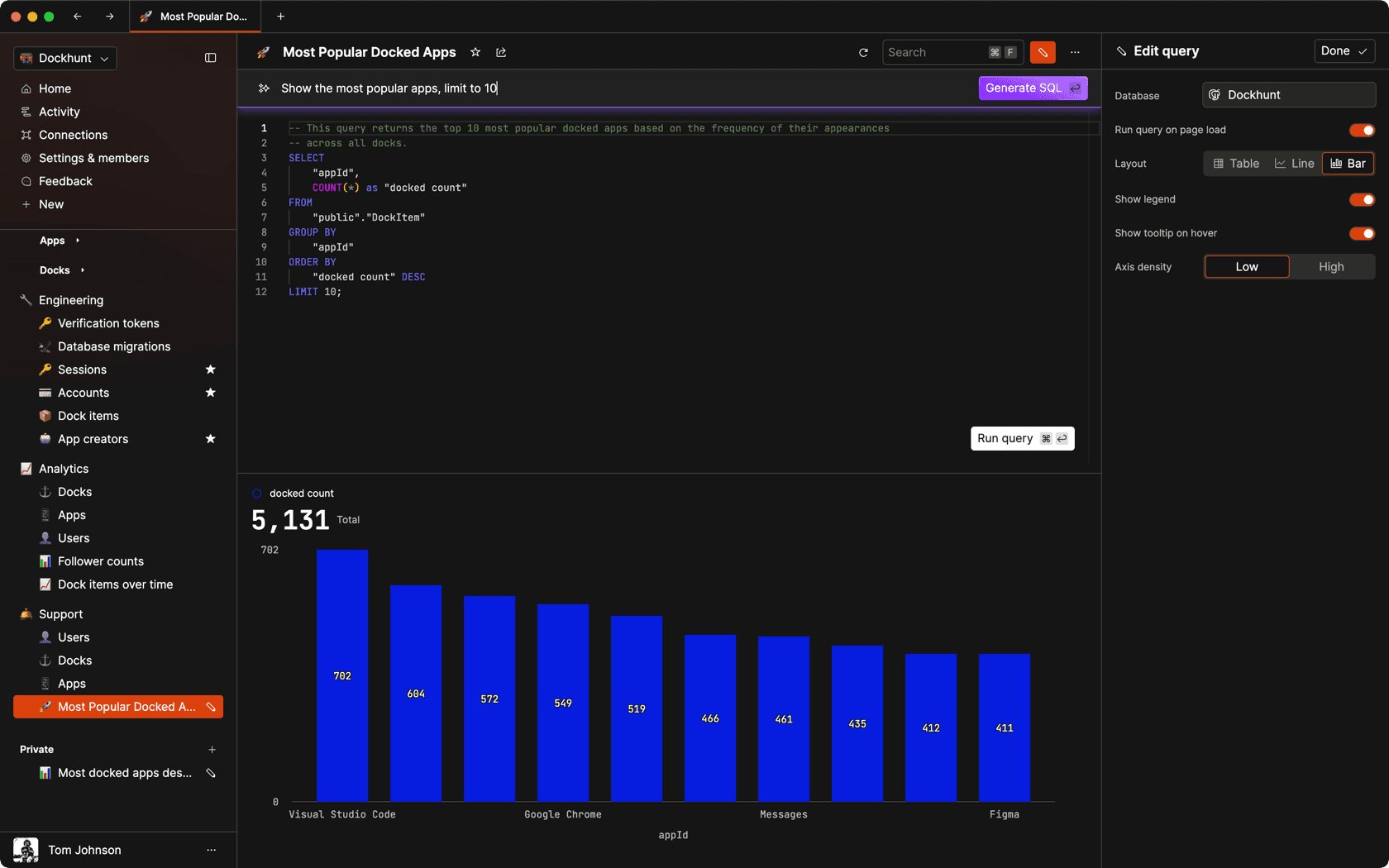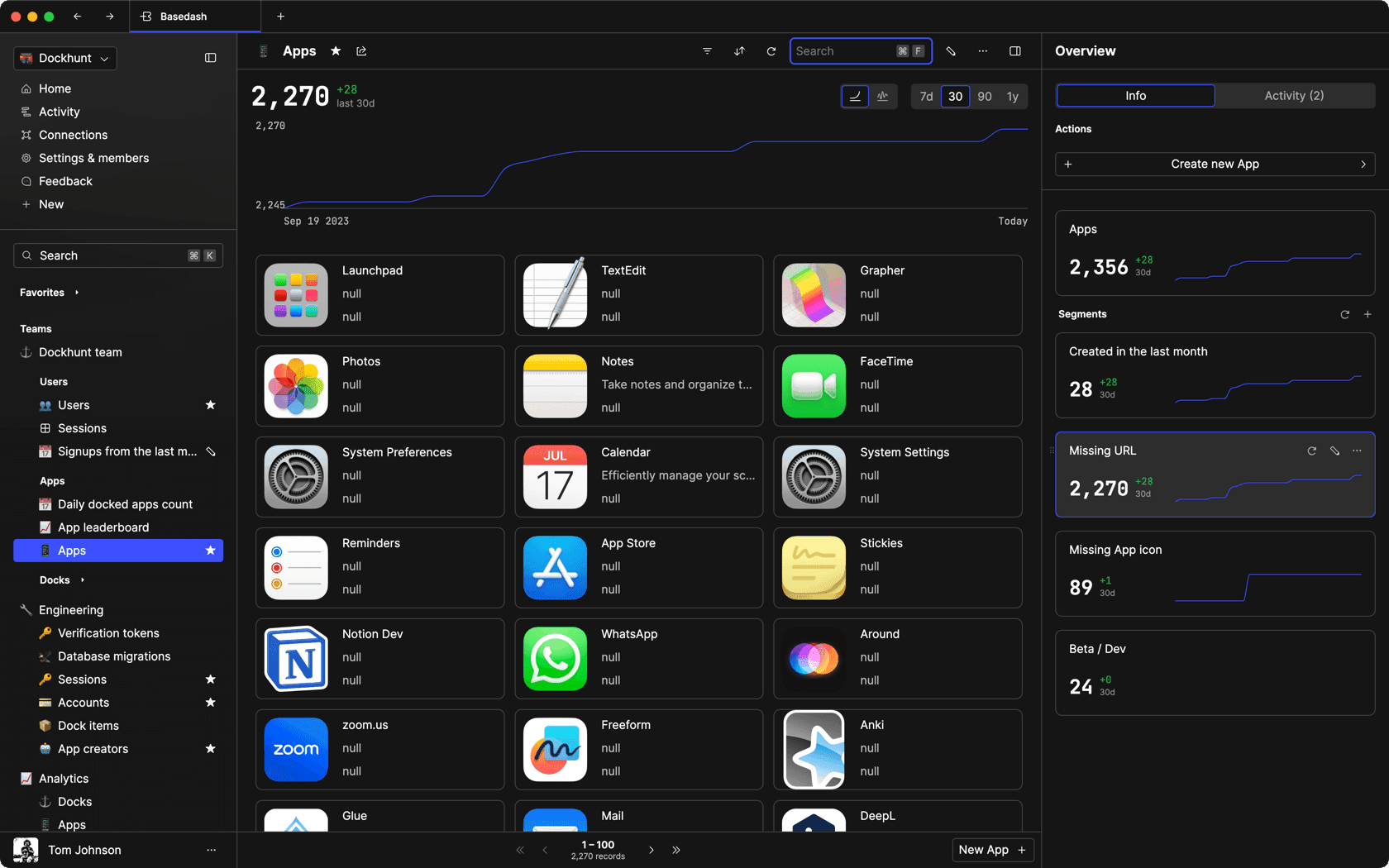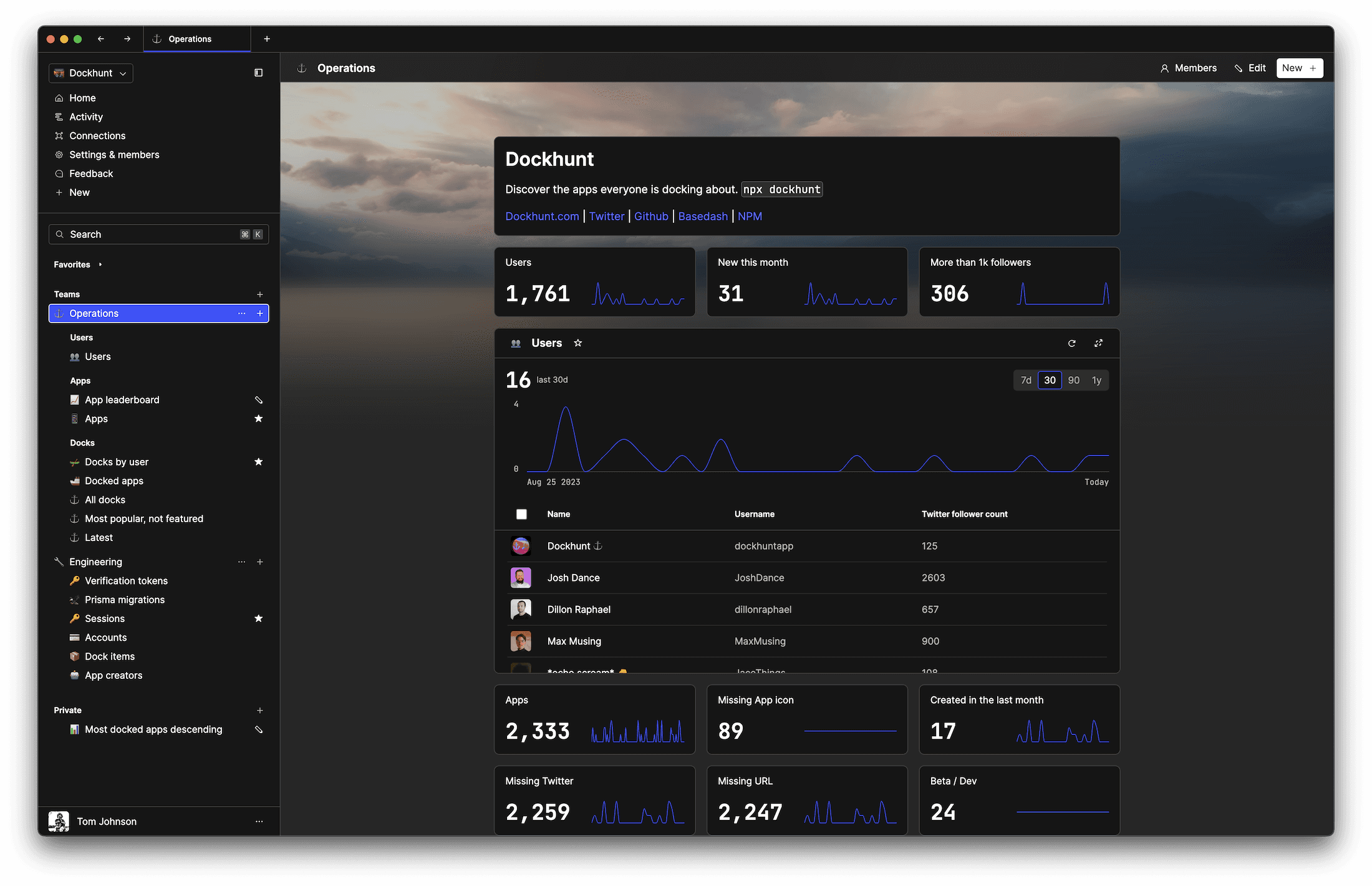# The Two Pointers Technique in JavaScriptself.__wrap_n=self.__wrap_n||(self.CSS&&CSS.supports("text-wrap","balance")?1:2);self.__wrap_b=(e,r,t)=>{t=t||document.querySelector(`[data-br="\${e}"]`);let n=t.parentElement,a=s=>t.style.maxWidth=s+"px";t.style.maxWidth="";let o,i=n.clientWidth,l=n.clientHeight,c=i/2-.25,f=i+.5;if(i){for(a(c),c=Math.max(t.scrollWidth,c);c+1<f;)o=Math.round((c+f)/2),a(o),n.clientHeight===l?f=o:c=o;a(f*r+i*(1-r))}t.__wrap_o||typeof ResizeObserver<"u"&&(t.__wrap_o=new ResizeObserver(()=>{self.__wrap_b(0,+t.dataset.brr,t)})).observe(n)};self.__wrap_n!=1&&self.__wrap_b(":Rj0pm:",1)

November 8, 2023

The two pointers technique is an efficient method used to solve problems involving sequences, such as arrays, by using two references to traverse the data structure in one pass. This approach can lead to more elegant and less resource-intensive solutions.

## Understanding the two pointers technique

The essence of this technique is to take two references—pointers—to elements in a structure like an array and move them towards or away from each other to analyze or manipulate the elements. The pointers could move from the opposite ends of the array towards the center, or they could start at the same position and move in the same direction, depending on the problem at hand.

## When to use two pointers

This technique comes in handy for problems that involve paired elements, like finding a pair that sums up to a particular value or merging two sorted arrays. It's especially useful when you need to minimize space complexity, often allowing for an in-place solution that doesn't require additional data structures.

## How two pointers work

### Opposite direction movement

```function findSumPair(arr, sum) {
let left = 0;
let right = arr.length - 1;

while (left < right) {
const currentSum = arr[left] + arr[right];
if (currentSum === sum) {
return [left, right];
} else if (currentSum < sum) {
left++;
} else {
right--;
}
}

return null;
}```

### Same direction movement

```function maxConsecutiveSum(arr, k) {
let maxSum = 0;
let tempSum = 0;
let start = 0;

for (let end = 0; end < arr.length; end++) {
tempSum += arr[end];
if (end >= k - 1) {
maxSum = Math.max(maxSum, tempSum);
tempSum -= arr[start];
start++;
}
}

return maxSum;
}```

## Examples of two pointers

### Removing duplicates from sorted array

```function removeDuplicates(arr) {
if (arr.length === 0) return 0;

let i = 0;
for (let j = 1; j < arr.length; j++) {
if (arr[j] !== arr[i]) {
i++;
arr[i] = arr[j];
}
}

return i + 1;
}```

### Reversing a string in place

```function reverseString(s) {
let left = 0;
let right = s.length - 1;

while (left < right) {
[s[left], s[right]] = [s[right], s[left]];
left++;
right--;
}
}```

## Benefits and considerations

Using the two pointers technique simplifies code and reduces the need for nested loops, thus cutting down on time complexity. It's important to consider this approach when dealing with linear data structures and when optimizing for efficiency. However, it requires a good understanding of the problem's constraints to apply effectively.

# Ship faster, worry less with Basedash

You're busy enough with product work to be weighed down building, maintaining, scoping and developing internal apps and admin panels. Forget all of that, and give your team the admin panel that you don't have to build. Launch in less time than it takes to run a standup.

You're busy enough with product work to be weighed down building, maintaining, scoping and developing internal apps and admin panels. Forget all of that, and give your team the admin panel that you don't have to build. Launch in less time than it takes to run a standup.

You're busy enough with product work to be weighed down building, maintaining, scoping and developing internal apps and admin panels. Forget all of that, and give your team the admin panel that you don't have to build. Launch in less time than it takes to run a standup.### Dashboards and charts

Effortlessly make charts and create a space for your team to work together towards shared goals and metrics.

### SQL composer with AISOC II
Type 2 Certified

### Features

Proudly carbon-neutral.SOC II
Type 2 Certified

### Features

Proudly carbon-neutral.SOC II
Type 2 Certified